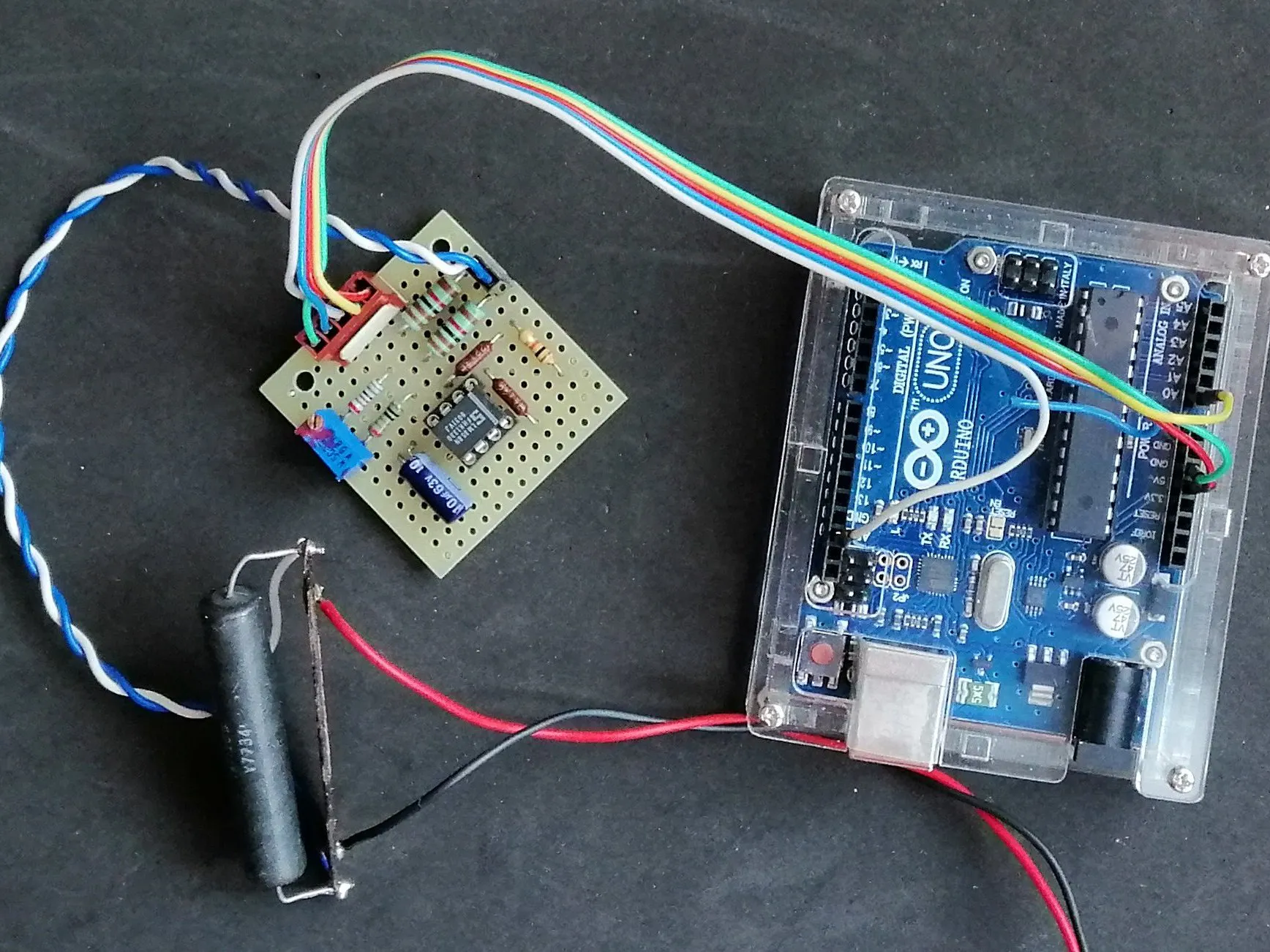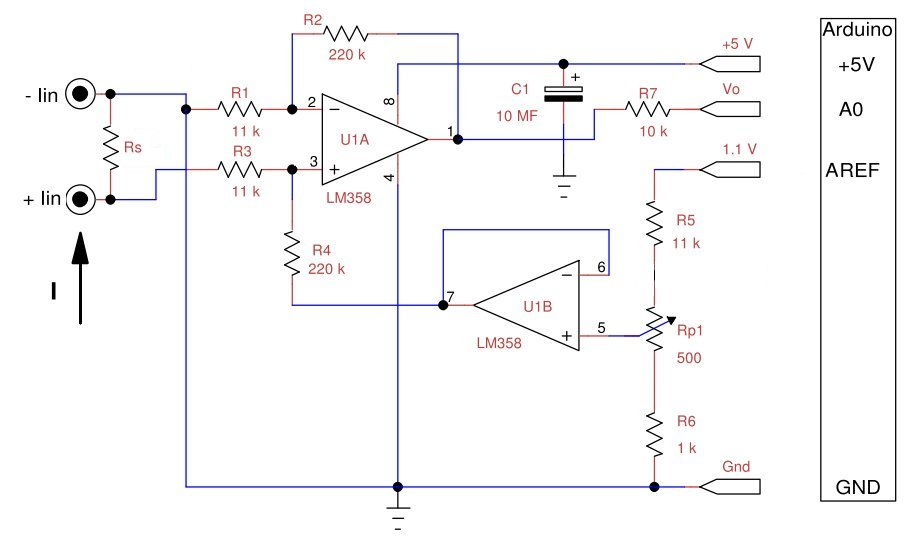Project tutorialA simple circuit for measuring electrical current with Arduino.

• 2,797 views
• 9 respects

## Necessary tools and machinesSoldering iron (generic)Wire Stripper & Cutter, 18-10 AWG / 0.75-4mm² Capacity Wires

## Apps and online services

There are several breakout boards available on the market ready to measure the current with Arduino, but for those who love self-construction I suggest to carry out this project. It is a fairly accurate current sensor while using common components.

The most accurate system for current measurement is to use a shunt, i.e. a resistor with very low resistance compared to that of the load and the generator. The voltage drop on this resistor, according to Ohm's law, is proportional to the current that passes through it.

Of course today we also find on the market sensors based on the Hall effect, that are devices that measure the magnetic field generated by the current passing through a wire for the well-known Ampere’s law, but these semiconductor sensors do not have comparable stability and precision.

To measure the voltage drop I used the shunt connected in a low-side way, i.e. towards ground and a differential amplifier.

The operational amplifiers, due to the saturation of the output stage, fail to give a zero at the output in the case of single power supply. I solved this problem by adding a constant Vsh = 100mV which I then delete via software. The output of differential amplifier U1a is:

Vo = (Vi+ - Vi-)×R2/R1+Vsh

The second operational U1b, connected as voltage follower, generates the voltage Vsh by means of a divider on the Arduino Aref.

For correct operation the following relations must be respected: R1=R3 and R2=R4 with very low tolerances. The ADC converter has a full scale of 1100mV and I take 100 out of it to make the zero, so I can have 1000 mV for the measurement, this means that the maximum input voltage must be 1000/20 = 50 mV. To have a sensitivity of 75 mV, suitable for many commercial shunts, it is sufficient to set R1 = R3 = 15kΩ and R2 = R4 = 200kΩ, standard and easily available values.

To test this circuit I used a 0.1 Ω shunt resistor and a 0.1 A current source.

A more detailed description of this project can be found in my blog: http://ardupiclab.blogspot.it/.

The following figure shows the layout and the connections of the components of my prototype, made on a small pre-drilled PCB board.

## Code

##### ArduAmmeter.inoArduino
The example program is very simple, the values used are relative to my system. To calibrate the Rp1 trimmer, just run the program and turn the trimmer to read zero on the serial monitor with zero input current. If it is not possible to zero the trimmer, it is necessary to slightly modify the Nsh constant to be subtracted from the measurement.
The constant mVtomA is derived from experimental measurements using a good precision ammeter, a power supply and some resistive loads such as car bulbs or wire resistors of adequate power.
```/* program ArduAmmeter.ino Arduino current meter
Giovanni Carrera, rev. 11/07/2019 */

float NtomV;
const float VREF = 1095;// in mV, this value can be read on VREF pin
const int Nsh = 98;// shift value corresponding to about 100 mV

void setup() {
Serial.begin(9600);
analogReference(INTERNAL); // internal ADC reference input = 1100 mV
NtomV = VREF/1023;// constant of conversion into millivolts
}

void loop() {
int val = analogRead(A0)-Nsh;// read the current sensor and remove the shift
float mvolt = NtomV*val;// convert to millivolt
float mamp = mvolt/2.17;// convert to milliampere
Serial.print("Vo = ");
Serial.print(mvolt,1);
Serial.print(" mV - Current = ");
Serial.print(mamp,0);
Serial.println(" mA");
delay(1000);
}
```

## SchematicsJuly 16, 2019

#### Members who respect this project

See similar projects
you might like

#### 2 Factor Authentication for ATM Machine Project

Project tutorial by Brijesh Singh

• 1,282 views
• 4 respects

#### Micro Soldering Station

Project tutorial by Paulo Bruckmann

• 18,846 views
• 52 respects

#### Integrated Solar ChargeController, Inverter, PowerBank, Lamp

Project tutorial by Shahariar

• 13,276 views
• 45 respects

Project tutorial by Giovanni Carrera

• 7,488 views
• 8 respects